# Chapter_4 (Advanced counting methods) (1).pdf

Mar. 24, 2023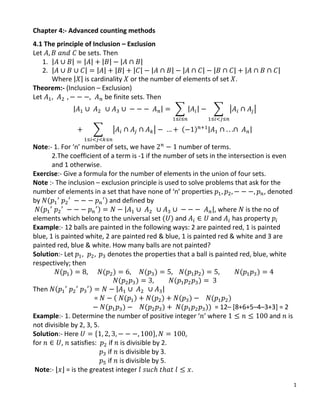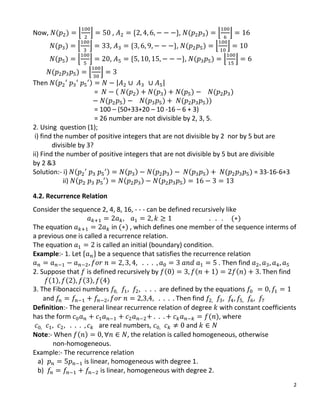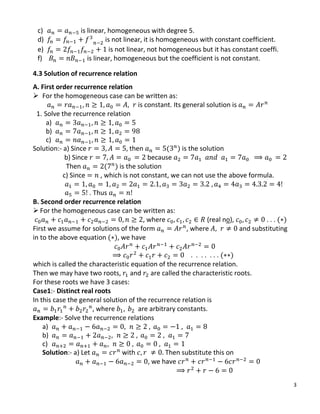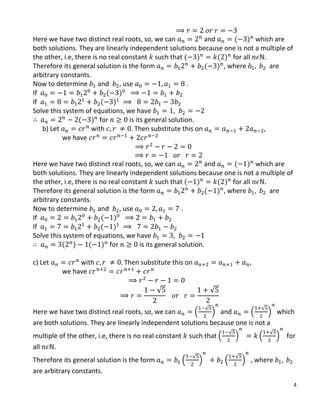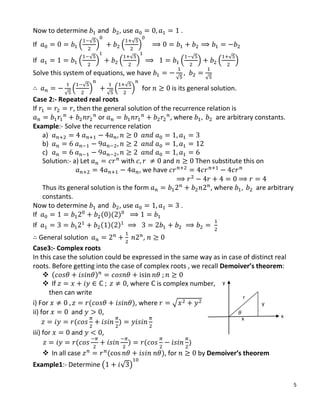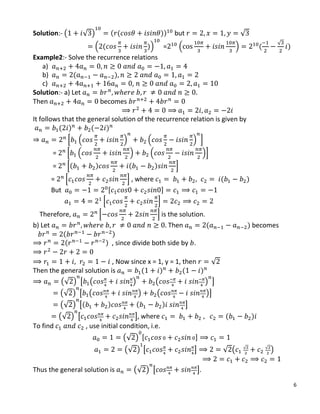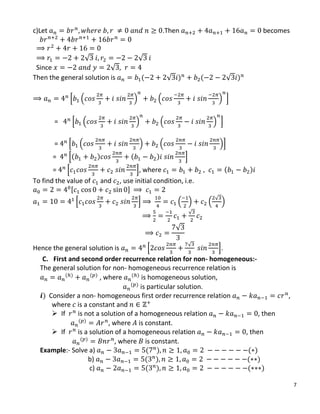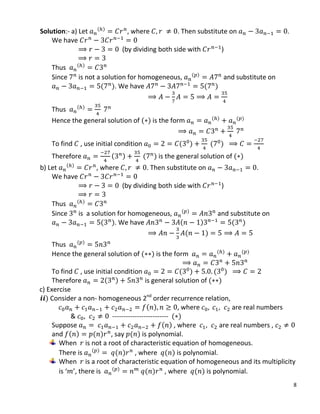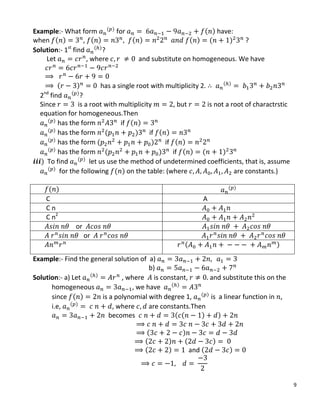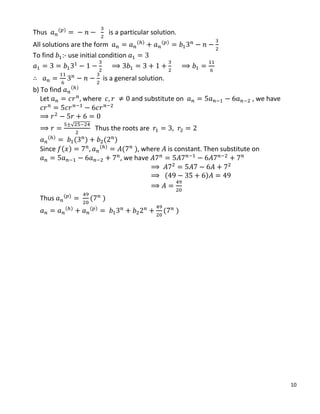Upcoming SlideShare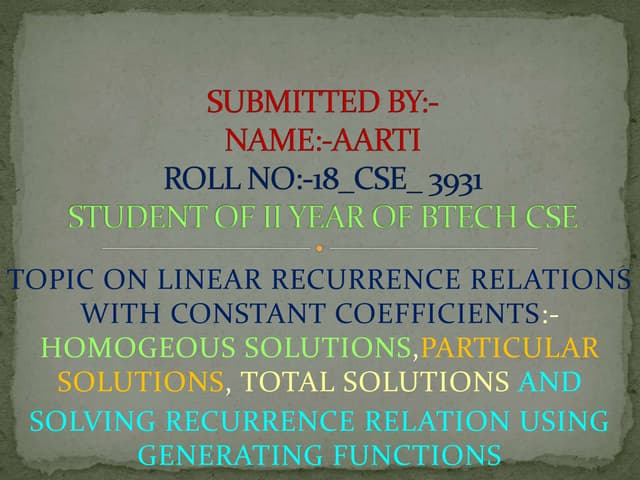LINEAR RECURRENCE RELATIONS WITH CONSTANT COEFFICIENTS
1 of 10

### Chapter_4 (Advanced counting methods) (1).pdf

1. 1 Chapter 4:- Advanced counting methods 4.1 The principle of Inclusion – Exclusion Let be sets. Then 1. | | | | | | | | 2. | | | | | | | | | | | | | | | | Where | | is cardinality or the number of elements of set . Theorem:- (Inclusion – Exclusion) Let be finite sets. Then | | ∑ | | ∑ | | ∑ | | ( ) | | Note:- 1. For ‘n’ number of sets, we have number of terms. 2.The coefficient of a term is -1 if the number of sets in the intersection is even and 1 otherwise. Exercise:- Give a formula for the number of elements in the union of four sets. Note :- The inclusion – exclusion principle is used to solve problems that ask for the number of elements in a set that have none of ‘n’ properties , denoted by ( ) and defined by ( ) | |, where is the no of elements which belong to the universal set ( ) and and has property Example:- 12 balls are painted in the following ways: 2 are painted red, 1 is painted blue, 1 is painted white, 2 are painted red & blue, 1 is painted red & white and 3 are painted red, blue & white. How many balls are not painted? Solution:- Let denotes the properties that a ball is painted red, blue, white respectively; then ( ) ( ) ( ) ( ) ( ) ( ) ( ) Then ( ) | | = ( ( ) ( ) ( ) ( ) ( ) ( ) ( )) = 12– [8+6+5–4–3+3] = 2 Example:- 1. Determine the number of positive integer ‘ ’ where and is not divisible by 2, 3, 5. Solution:- Here * + , for , satisfies: if is divisible by 2. if is divisible by 3. if is divisible by 5. Note:- ⌊ ⌋ = is the greatest integer .
2. 2 Now, ( ) ⌊ ⌋ , * +, ( ) ⌊ ⌋ ( ) ⌊ ⌋ , * +, ( ) ⌊ ⌋ ( ) ⌊ ⌋ , * +, ( ) ⌊ ⌋ ( ) ⌊ ⌋ Then ( ) | | = ( ( ) ( ) ( ) ( ) ( ) ( ) ( )) = 100 – (50+33+20 – 10 -16 – 6 + 3) = 26 number are not divisible by 2, 3, 5. 2. Using question (1); i) find the number of positive integers that are not divisible by 2 nor by 5 but are divisible by 3? ii) Find the number of positive integers that are not divisible by 5 but are divisible by 2 &3 Solution:- i) ( ) ( ) ( ) ( ) ( ) = 33-16-6+3 ii) ( ) ( ) ( ) 4.2. Recurrence Relation Consider the sequence 2, 4, 8, 16, - - - can be defined recursively like ( ) The equation in ( ) , which defines one member of the sequence interms of a previous one is called a recurrence relation. The equation is called an initial (boundary) condition. Example:- 1. Let * + be a sequence that satisfies the recurrence relation . Then find 2. Suppose that is defined recursively by ( ) ( ) ( ) Then find ( ) ( ) ( ) ( ) 3. The Fibonacci numbers are defined by the equations and Then find Definition:- The general linear recurrence relation of degree with constant coefficients has the form ( ), where are real numbers, and Note:- When ( ) , the relation is called homogeneous, otherwise non-homogeneous. Example:- The recurrence relation a) is linear, homogeneous with degree 1. b) is linear, homogeneous with degree 2.
3. 3 c) is linear, homogeneous with degree 5. d) is not linear, it is homogeneous with constant coefficient. e) is not linear, not homogeneous but it has constant coeffi. f) is linear, homogeneous but the coefficient is not constant. 4.3 Solution of recurrence relation A. First order recurrence relation  For the homogeneous case can be written as: is constant. Its general solution is 1. Solve the recurrence relation a) b) c) Solution:- a) Since then ( ) is the solution b) Since because Then ( ) is the solution c) Since , which is not constant, we can not use the above formula. . Thus B. Second order recurrence relation  For the homogeneous case can be written as: , where (real no), . . . ( ) First we assume for solutions of the form , where and substituting in to the above equation ( ), we have . . . . . . . ( ) which is called the characteristic equation of the recurrence relation. Then we may have two roots, and are called the characteristic roots. For these roots we have 3 cases: Case1:- Distinct real roots In this case the general solution of the recurrence relation is , where are arbitrary constants. Example:- Solve the recurrence relations a) b) c) Solution:- a) Let with Then substitute this on , we have
4. 4 Here we have two distinct real roots, so, we can and ( ) which are both solutions. They are linearly independent solutions because one is not a multiple of the other, i.e, there is no real constant such that ( ) ( ) for all Therefore its general solution is the form ( ) , where are arbitrary constants. Now to determine and , use . If ( ) If ( ) Solve this system of equations, we have ( ) for is its general solution. b) Let with Then substitute this on , we have Here we have two distinct real roots, so, we can and ( ) which are both solutions. They are linearly independent solutions because one is not a multiple of the other, i.e, there is no real constant such that ( ) ( ) for all Therefore its general solution is the form ( ) , where are arbitrary constants. Now to determine and , use . If ( ) If ( ) Solve this system of equations, we have ( ) ( ) for is its general solution. c) Let with Then substitute this on we have √ √ Here we have two distinct real roots, so, we can . √ / and . √ / which are both solutions. They are linearly independent solutions because one is not a multiple of the other, i.e, there is no real constant such that . √ / . √ / for all Therefore its general solution is the form . √ / . √ / , where are arbitrary constants.
5. 5 Now to determine and , use . If . √ / . √ / If . √ / . √ / . √ / . √ / Solve this system of equations, we have √ √ √ . √ / √ . √ / for is its general solution. Case 2:- Repeated real roots If , then the general solution of the recurrence relation is or , where are arbitrary constants. Example:- Solve the recurrence relation a) b) c) Solution:- a) Let with and Then substitute this on , we have Thus its general solution is the form , where are arbitrary constants. Now to determine and , use . If ( )( ) If ( )( ) General solution , Case3:- Complex roots In this case the solution could be expressed in the same way as in case of distinct real roots. Before getting into the case of complex roots , we recall Demoiver’s theorem:  ( )  If , where is complex number, then can write i) For ( ), where √ ii) for and , ( ) iii) for and , ( ) ( )  In all case ( ), for by Demoiver’s theorem Example1:- Determine ( √ ) y r x 𝜃 x y
6. 6 Solution:- ( √ ) ( ( )) but √ . ( )/ = . / ( √ ) Example2:- Solve the recurrence relations a) b) ( ) c) , Solution:- a) Let Then becomes It follows that the general solution of the recurrence relation is given by ( ) ( ) 0 . / . / 1 = 0 . / . /1 = 0( ) ( ) 1 = 0 1 , where ( ) But , - 0 1 Therefore, 0 1 is the solution. b) Let Then ( ) becomes ( ) ( ) , since divide both side by . , Now since x = 1, y = 1, then √ Then the general solution is ( ) ( ) (√ ) [ ( ) ( ) ] (√ ) [ ( ) ( )] (√ ) [( ) ( ) ] (√ ) [ ], where ( ) To find , use initial condition, i.e. (√ ) , - (√ ) [ ] √ ( √ √ ) Thus the general solution is (√ ) [ ].
7. 7 c)Let .Then becomes √ √ Since √ Then the general solution is ( √ ) ( √ ) 0 . / . / 1 = 0 . / . / 1 = 0 . / . /1 = 0( ) ( ) 1 = 0 1, where ( ) To find the value of and , use initial condition, i.e. , - 0 1 . / . √ / √ √ Hence the general solution is 0 √ 1. C. First and second order recurrence relation for non- homogeneous:- The general solution for non- homogeneous recurrence relation is ( ) ( ) , where ( ) is homogeneous solution, ( ) is particular solution. ) Consider a non- homogeneous first order recurrence relation , where is a constant and  If is not a solution of a homogeneous relation , then ( ) , where is constant.  If is a solution of a homogeneous relation , then ( ) , where is constant. Example:- Solve a) ( ) ( ) b) ( ) ( ) c) ( ) ( )
8. 8 Solution:- a) Let ( ) , where . Then substitute on . We have (by dividing both side with ) Thus ( ) Since is not a solution for homogeneous, ( ) and substitute on ( ). We have ( ) Thus ( ) Hence the general solution of ( ) is the form ( ) ( ) To find , use initial condition ( ) ( ) Therefore ( ) ( ) is the general solution of ( ) b) Let ( ) , where . Then substitute on . We have (by dividing both side with ) Thus ( ) Since is a solution for homogeneous, ( ) and substitute on ( ). We have ( ) ( ) ( ) Thus ( ) Hence the general solution of ( ) is the form ( ) ( ) To find , use initial condition ( ) ( ) Therefore ( ) is general solution of ( ) c) Exercise ) Consider a non- homogeneous 2nd order recurrence relation, ( ) , where are real numbers & ------------------------- ( ) Suppose ( ) , where are real numbers , and ( ) ( ) , say ( ) is polynomial. When is not a root of characteristic equation of homogeneous. There is ( ) ( ) , where ( ) is polynomial. When is a root of characteristic equation of homogeneous and its multiplicity is ‘ ’, there is ( ) ( ) , where ( ) is polynomial.
9. 9 Example:- What form ( ) for ( ) have: when ( ) , ( ) ( ) ( ) ( ) ? Solution:- 1st find ( ) ? Let , where and substitute on homogeneous. We have ( ) has a single root with multiplicity 2. ( ) 2nd find ( ) ? Since is a root with multiplicity , but is not a root of charactrstic equation for homogeneous.Then ( ) has the form if ( ) ( ) has the form ( ) if ( ) ( ) has the form ( ) if ( ) ( ) has the form ( ) if ( ) ( ) ) To find ( ) let us use the method of undetermined coefficients, that is, assume ( ) for the following ( ) on the table: (where are constants.) ( ) ( ) C A C n C n2 or or ( ) Example:- Find the general solution of a) b) Solution:- a) Let ( ) , where is constant, and substitute this on the homogeneous , we have ( ) since ( ) is a polynomial with degree 1, ( ) is a linear function in , i.e, ( ) , where are constants.Then becomes ( ( ) ) ( ) ( ) ( ) ( ) and ( )
10. 10 Thus ( ) is a particular solution. All solutions are the form ( ) ( ) To find :- use initial condition is a general solution. b) To find ( ) Let where and substitute on , we have √ Thus the roots are ( ) ( ) ( ) Since ( ) ( ) ( ), where is constant. Then substitute on , we have ( ) Thus ( ) ( ) ( ) ( ) ( )